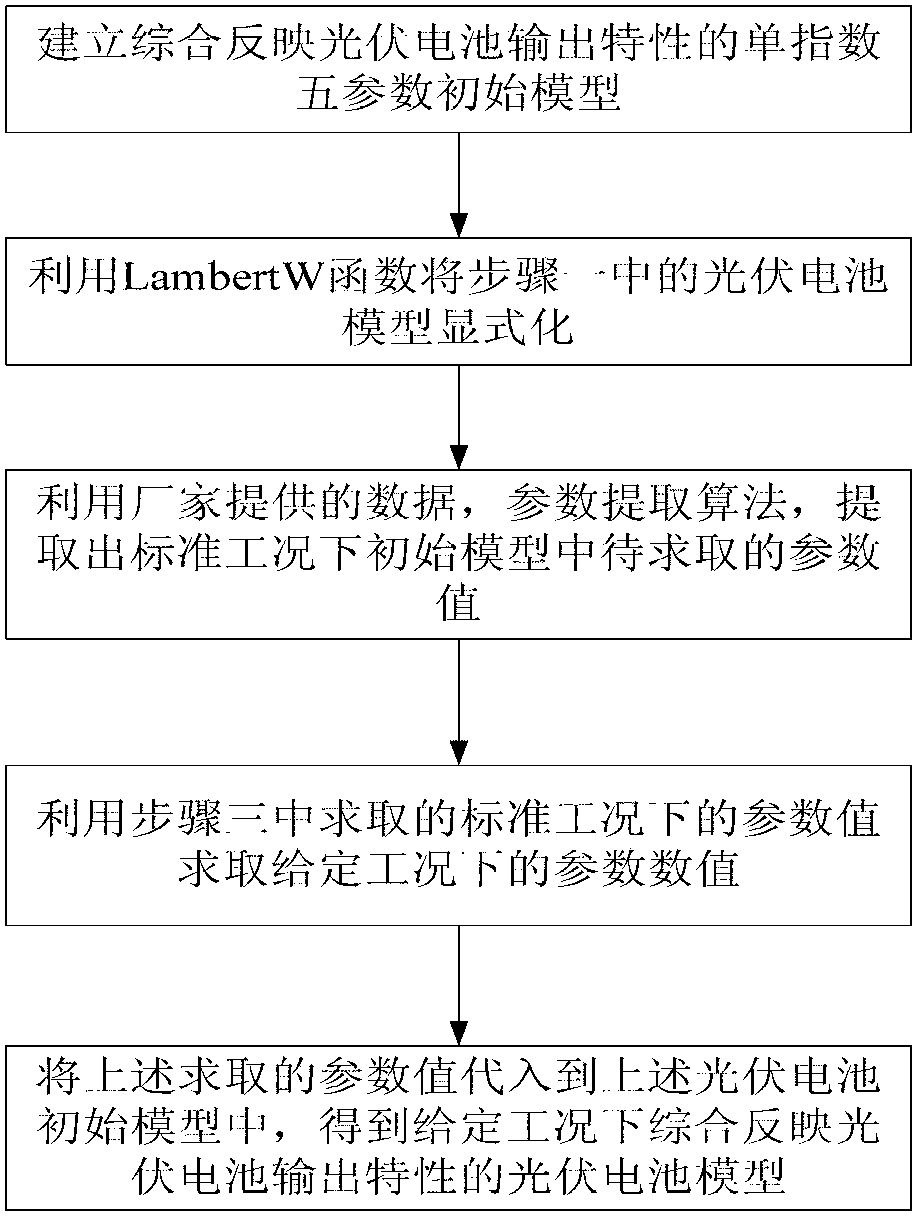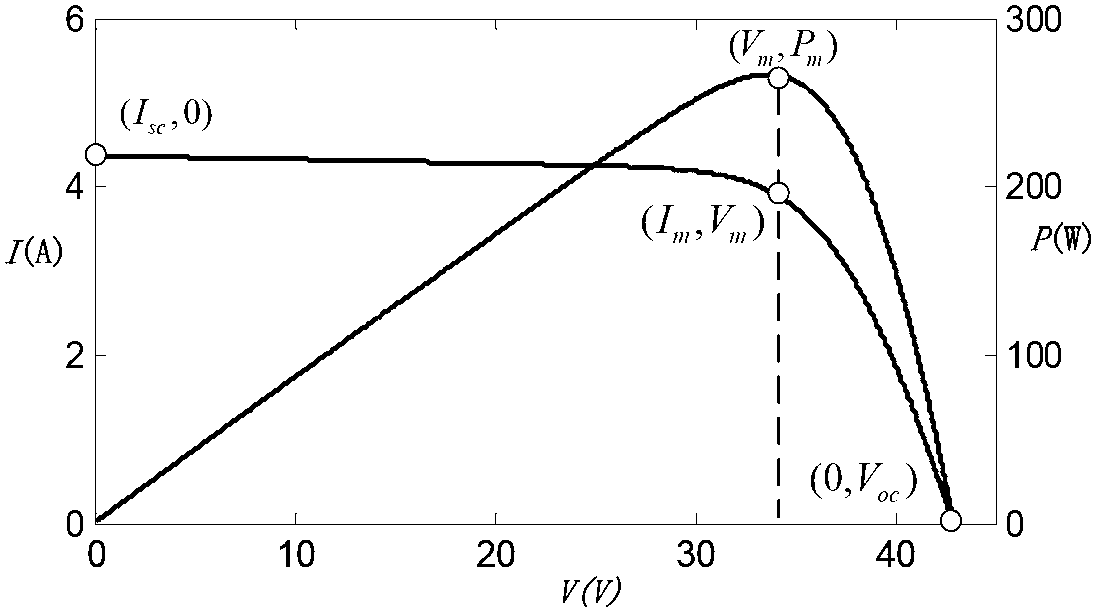# Photovoltaic cell output characteristic modeling method

## A photovoltaic cell and output characteristic technology, applied in the direction of electrical digital data processing, special data processing applications, instruments, etc., can solve problems such as large data and inaccurate photovoltaic cell models

Inactive Publication Date: 2013-03-27
NORTH CHINA ELECTRIC POWER UNIV (BAODING)
3 Cites 29 Cited by

## AI-Extracted Technical Summary

### Problems solved by technology

 Aiming at the problems mentioned in the background technology that the existing photovoltaic cell model is inaccurate, too much data is...
View more

## Abstract

The invention discloses a photovoltaic cell output characteristic modeling method in the technical field of photovoltaic power generation. According to the technical scheme, the method comprises the following the steps of: establishing a single exponential parameter initial model which comprehensively reflects photovoltaic cell output characteristics; enabling the single exponential parameter initial model to be explicit by utilizing a Lambert W function so as to obtain a photovoltaic cell explicit model; calculating a to-be-calculated parameter value in the initial model under a standard working condition by utilizing data provided by manufacturers and a parameter calculating algorithm; and calculating a parameter value under a given working condition by utilizing the parameter value under the standard working condition and a parameter transformation algorithm; and substituting the calculated parameter value into the photovoltaic cell explicit model, thereby obtaining the photovoltaic cell model which comprehensively reflects photovoltaic cell output characteristics under the given working condition. The photovoltaic cell output characteristic modeling method provided by the invention has the beneficial benefits that the established photovoltaic cell model is accurate; data of manufacturers are only utilized during the parameter calculating process, and the parameter calculating algorithm is simple, and is wide in application range; and the explicit model is simple and convenient to use.

Application Domain

Special data processing applications

Technology Topic

Photovoltaic power generationExplicit model +5

## Image

•••## Examples

• Experimental program(1)

### Example Embodiment

 The preferred embodiments will be described in detail below in conjunction with the accompanying drawings. It should be emphasized that the following description is only exemplary and not intended to limit the scope of the invention and its application.
 The specific steps of the modeling method are:
 Step 1: Establish a single exponential 5-parameter initial model that comprehensively reflects the output characteristics of photovoltaic cells, wherein the 5 parameters in the above initial model are all values ​​to be obtained.
 The equivalent circuit of a single exponential 5-parameter model of a photovoltaic cell is as figure 2 shown, according to figure 2 Available:
 I = I pv - I 0 [ exp ( V + R s I aV t ) - 1 ] - V + R s I R p - - - ( 1 )
 Among them: I pv is the photogenerated current; I 0 is the reverse saturation leakage current flowing through the diode; V t =N s kT/q,N s is the number of single cells contained in the photovoltaic cell module, k is the Boltzmann constant: 1.38×10 -23 J/K, T is the battery temperature, q is the unit charge, 1.6×10 -19 C; R s and R p are the equivalent series resistance and parallel resistance; a is the ideality factor; I and V are the output current and output voltage of the photovoltaic cell, respectively.
 Step 2: Use the Lambert W function to make the photovoltaic cell model explicit in step 1 to obtain an explicit photovoltaic cell model. The output current (voltage) can be obtained directly by inputting a given voltage (current).
 It can be seen that formula (1) is an implicit transcendental equation about I and V, which is inconvenient to solve, so the Lambert W function is used to make it explicit:
 I = R p ( I pv + I 0 ) - V R s + R p - aV t R s W ( Y ) - - - ( 2 )
 V=R p (I pv +I 0 -I)-IR s -aV t W(Z) (3)
 Among them, W(Y) and W(Z) are both Lambert W functions, satisfying the property W(X)exp(W(X))=X
 Y = R s R p I 0 aV t ( R s + R p ) exp [ R p ( R s I pv + R s I 0 + V ) aV t ( R s + R p ) ]
 Z = R p I 0 aV t exp ( R p ( I pv + I 0 - I ) aV t )
 It should be noted that explicitization refers to equivalently transforming the photovoltaic cell model in step 1 into a simple and easy-to-apply model. There is no simplification of the model.
 Step 3: Use the data provided by the manufacturer and the parameter calculation algorithm to extract the parameter values ​​to be obtained in the initial model under standard working conditions.
 Usually, photovoltaic cell manufacturers only provide users with standard operating conditions (irradiance S=1000W/m 2 , battery temperature T=25°C), photovoltaic cell short-circuit current I sc，n , open circuit voltage V oc，n , maximum power point current I m，n and voltage V m,n (Note: The parameter subscript with n indicates the corresponding value under standard working conditions, which will not be explained below).
 Correspondingly, the short-circuit point V=0, I=I sc，n :
 I pv , n ≈ R p , n + R s , n R p . n I sc , n - - - ( 4 )
 Open circuit point V=V oc，n , I=0:
 I 0 , n = ( R p , n + R s , n ) I sc , n - V oc , n C 1 R p , n - - - ( 5 )
 Among them: C 1 =exp[V oc,n /(a n V t，n )]-1
 Maximum power point V=V max，n , I=I max，n :
 R p , n = V max , n + R s , n I max , n - C 1 V oc , n / C 2 ( C 2 - C 1 ) / C 2 I sc , n - I max , n - - - ( 6 )
 Among them: C 2 =exp[(V max，n +I max，n R s,n )/(a n V t，n )]-1;
 Another by image 3 The P-V characteristic curve of the photovoltaic cell shown:
 dP dV | V m = V m dI dV | V m + I m = 0 - - - ( 7 )
 Among them, P is the battery output power; V is the output voltage value; V m is the voltage value corresponding to the maximum output power; I m is the current value corresponding to the maximum output power;
 It is further deduced that:
 R p , n = V max , n - aV t , n + I max , n R s , n + V oc , n / C 1 ( C 1 + 1 ) / C 1 I sc , n + [ aV t , n / ( I max , n R s , n - V m , n ) - 1 ] I max , n - - - ( 8 )
 The above two explicit equations contain a total of 3 unknown parameters R p，n 、a n and R s，n , the analytical solution cannot be obtained directly, and the following algorithm can be used for optimal solution:
 min|R p1,n (R s，n , a n )-R p2，n (R s，n , a n )|
 s.t.a min ≤a n ≤a max R s，min s，n s,max (9)
 For photovoltaic cells of different materials, a n The typical value of is also different, and photovoltaic cells of different materials are combined in the algorithm a n The typical value of a min =1.0, a max =3.5; From a physical point of view, R s，n Describes the resistance of the photovoltaic cell substrate, the resistance of the diffusion layer, and the contact resistance between the grid line and the photovoltaic cell, so that R s,n Impossible to be less than 0, preferably R s，min =0; for general photovoltaic cells, R s,n Small, desirable R s，max =2.
 Step 4: Use the parameter values ​​under standard working conditions obtained in step 3 and the parameter conversion algorithm to obtain the parameter values ​​under given working conditions.
 Photovoltaic cell model parameters vary with irradiance and temperature. Photogenerated current is mainly affected by irradiance and temperature:
 I pv = [ I pv , n + K I ( T - T n ) ] S S n - - - ( 10 )
 Among them: K I is the current temperature coefficient; S n Irradiance under standard conditions.
 Diode reverse saturation leakage current is mainly affected by temperature:
 I 0 = I 0 , n ( T T n ) 3 exp [ qE g a n k ( 1 T n - 1 T ) ] - - - ( 11 )
 Among them: E g For the forbidden band width, different photovoltaic cell materials take different values ​​(eg: Si: 1.12eV, GaGs: 1.35eV).
 For a, R under normal working conditions s , R p , which can be obtained by the following formula:
 a=a n (12)
 R s =R s，n (13)
 R p = S S n × R p , n - - - ( 14 )
 When R is obtained under the standard working condition through the optimization algorithm p，n 、a n and R s,n After that, the corresponding parameter values ​​under general working conditions can be obtained through the above conversion formula. When the above algorithm is used to calculate the model parameters, the corresponding values ​​of the five parameters under any irradiance and temperature can be obtained through the corresponding conversion formulas after only one off-line calculation of the five parameters under the standard working condition of the photovoltaic cell, so that it can be easily It is convenient for real-time simulation of photovoltaic cells.
 Step 5: Substituting the parameter values ​​obtained above into the explicit model of the photovoltaic cell in step 2 to obtain a photovoltaic cell model that comprehensively reflects the output characteristics of the photovoltaic cell under a given working condition.
 The above is only a preferred embodiment of the present invention, but the scope of protection of the present invention is not limited thereto. Any person skilled in the art within the technical scope disclosed in the present invention can easily think of changes or Replacement should be covered within the protection scope of the present invention. Therefore, the protection scope of the present invention should be determined by the protection scope of the claims.

## PUM## Description & Claims & Application Information

We can also present the details of the Description, Claims and Application information to help users get a comprehensive understanding of the technical details of the patent, such as background art, summary of invention, brief description of drawings, description of embodiments, and other original content. On the other hand, users can also determine the specific scope of protection of the technology through the list of claims; as well as understand the changes in the life cycle of the technology with the presentation of the patent timeline. Login to view more.
Who we serve
• R&D Engineer
• R&D Manager
• IP Professional
Why Eureka